Function Repository Resource:

# BlockCellularAutomaton

Evolve a block cellular automaton

Contributed by: Wolfram Research
 ResourceFunction["BlockCellularAutomaton"][rule,{init,phase},t] generates a list of states and phases representing the evolution of the block cellular automaton with the specified rule from initial state init and initial phase for t steps. ResourceFunction["BlockCellularAutomaton"][rule,{init,phase}] gives the result of evolving {init,phase} for one step. ResourceFunction["BlockCellularAutomaton"][rule] is an operator form of BlockCellularAutomaton that affects one step of evolution. ResourceFunction["BlockCellularAutomaton"][rule,init,t] provides legacy support for previous input/output format, assuming phase=0, and returning a flat list of states with no phase information. ResourceFunction["BlockCellularAutomaton"][rule,init,t,phase] provides legacy support with an initial phase. ResourceFunction["BlockCellularAutomaton"][rule,init] provides legacy support for one step evolution assuming phase=0.

## Details

Possible forms for rule are:
 {in1→out1,in2→out2,…} A list of rules Dispatch[{in1→out1,…}] An optimized list of rules Association[in1→out1,…] A lookup dictionary {rule, dim} with rule any of the above, and dim equals block length
Specification by Association may lead to the fastest calculations, but it requires comprehensive list of inputs without any usage of pattern matching or RuleDelayed. Lists of rules may lead to slightly slower calculations, but are more flexible by allowing for pattern matching. The Dispatch usage should not be much slower than the Association usage.
ResourceFunction["BlockCellularAutomaton"] allows one extra rule specification {rule,{2,2}} for evolutions through two spatial dimensions, which alternate between phase values 0 and 1 with {2,2} block offset along the diagonal. This is sometimes referred to as the "Margolus neighborhood". More algorithm design regarding phases and offsets remains to be done to implement full dimensional generalization of ResourceFunction["BlockCellularAutomaton"].
Possible forms for time t are:
 t all steps 0 through t {t} a list containing only step t {{t}} step t alone {t1,t2} steps t1 through t2 {t1,t2,dt} steps t1,t1+dt, …
List items in the output of ResourceFunction["BlockCellularAutomaton"] take the form {statei, phasei}, where phase goes from 0,1,,dim-1 and dim is the fixed block length. To obtain an ArrayPlot of a time evolution, simply discard phase information by combining Map and First, as in examples given below.
The length of init should be commensurate with the block size dim.
Previous versions of this code used a different phase convention, phase ∈{1,2,…, dim}. Backwards compatible usage expects a phase in the previous convention and automatically subtracts one to convert to the present convention.

## Examples

### Basic Examples (4)

Evolve a block cellular automaton for three steps:

 In:=Out=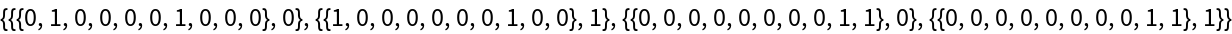Plot the same block cellular automaton evolving over 20 steps:

 In:=Out=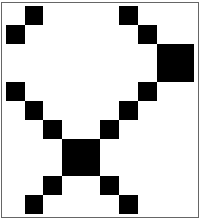Evolve a block cellular automaton for one step:

 In:=Out=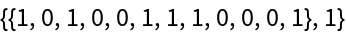Find the state of a block cellular automaton after 100 time steps:

 In:=Out=Use the operator form to evolve a block cellular automaton by one step:

 In:=Out=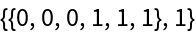Apply the same operator to all possible length-6 inputs and produce a state transition Graph:

 In:=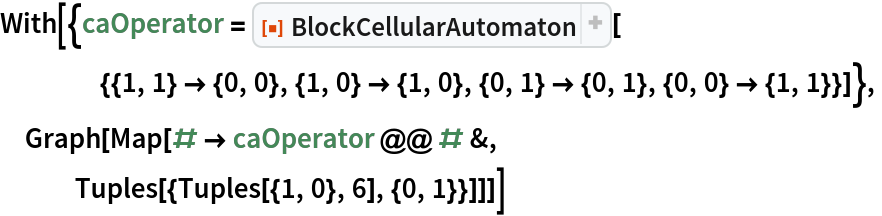Out=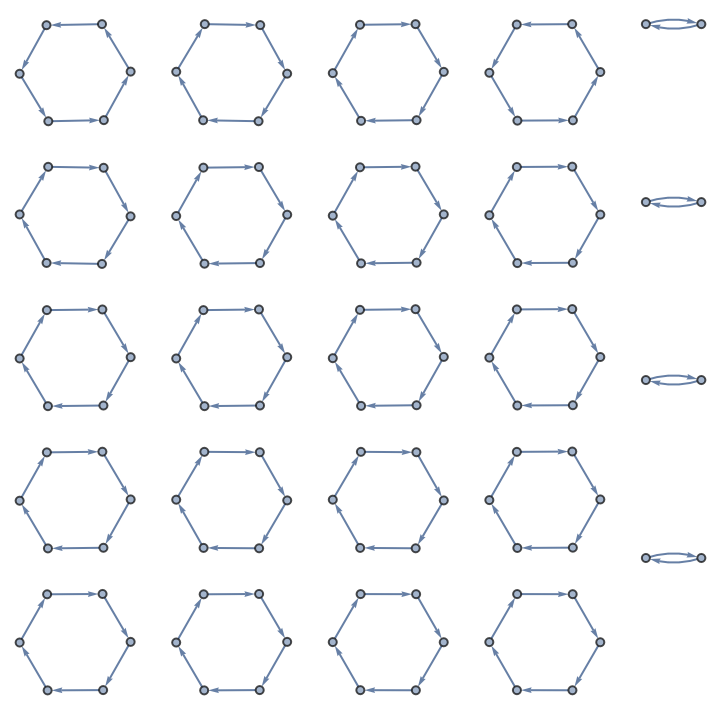### Scope (2)

BlockCellularAutomaton allows block lengths greater than 2, and variable rules:

 In:=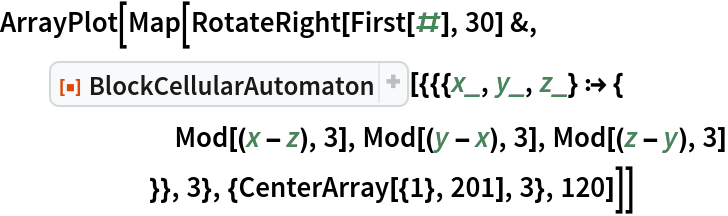Out=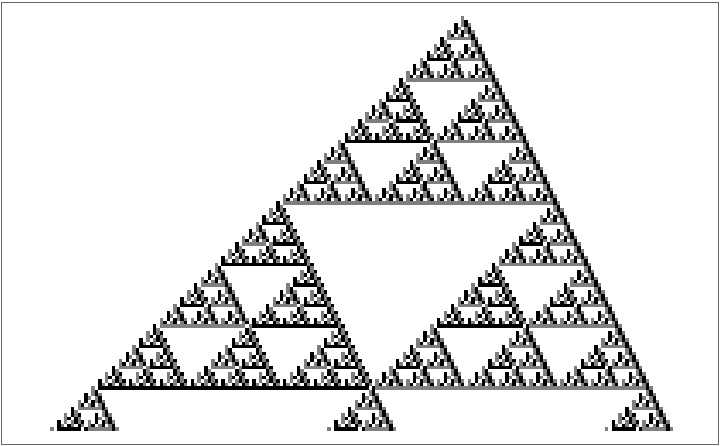BlockCellularAutomaton has a special use case for rules on the Margolus neighborhood such as this "billiards" example:

 In:=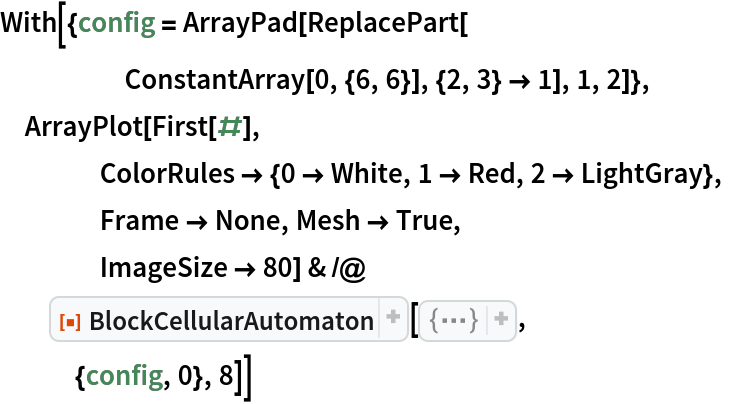Out=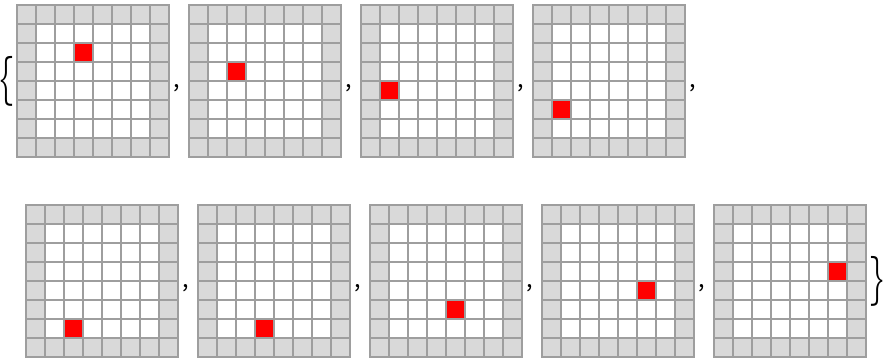### Applications (1)

Plot a block cellular automaton from A New Kind of Science:

 In:=Out=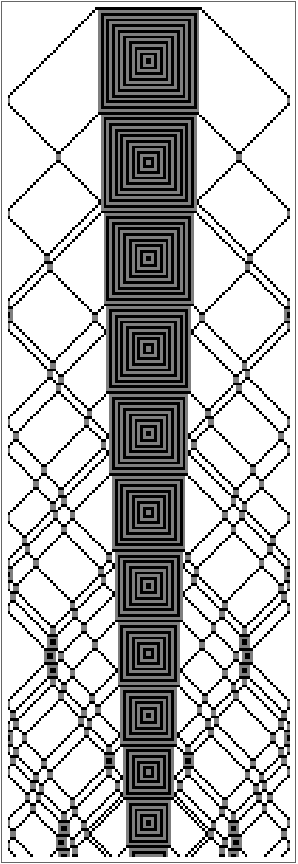### Properties and Relations (3)

BlockCellularAutomaton is backwards compatible with previous input/output forms:

 In:=Out=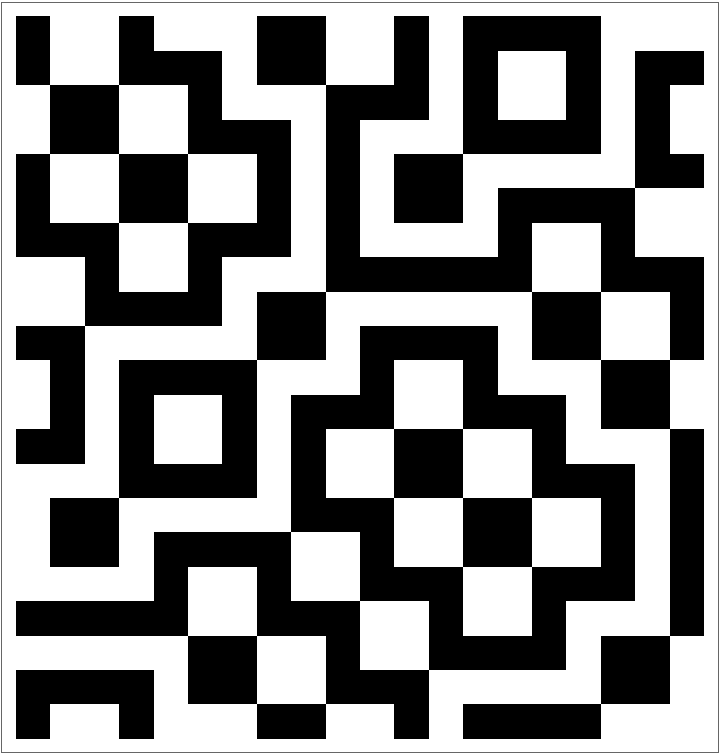Backwards compatibility also allows initializing with a phase:

 In:=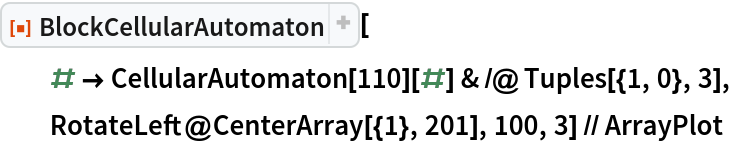Out=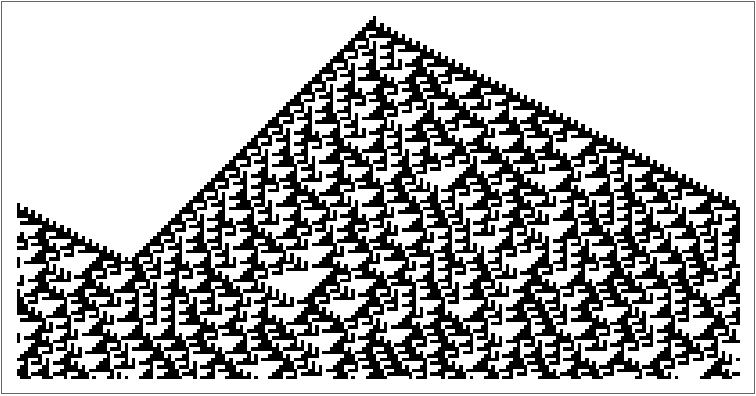Backwards compatibility also allows one-step evolution assuming initial phase=0:

 In:=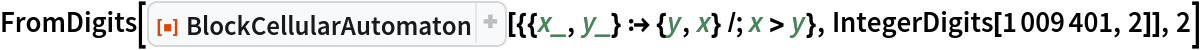Out=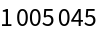### Possible Issues (2)

Block size must divide the length of the initial condition:

 In:=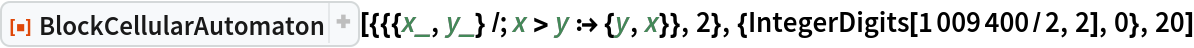Out=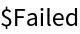Rules must have equal block size:

 In:=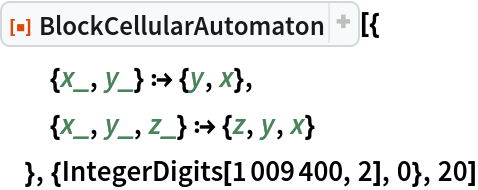Out=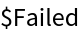### Neat Examples (2)

Find a periodic solution of the "billiards" block cellular automaton shown above and plot the cycle:

 In:=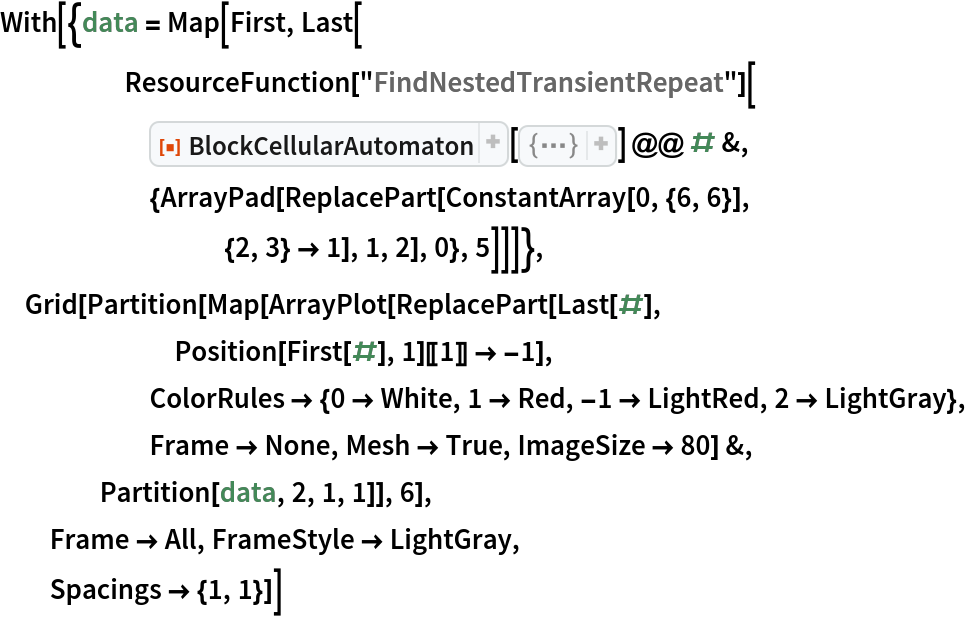Out=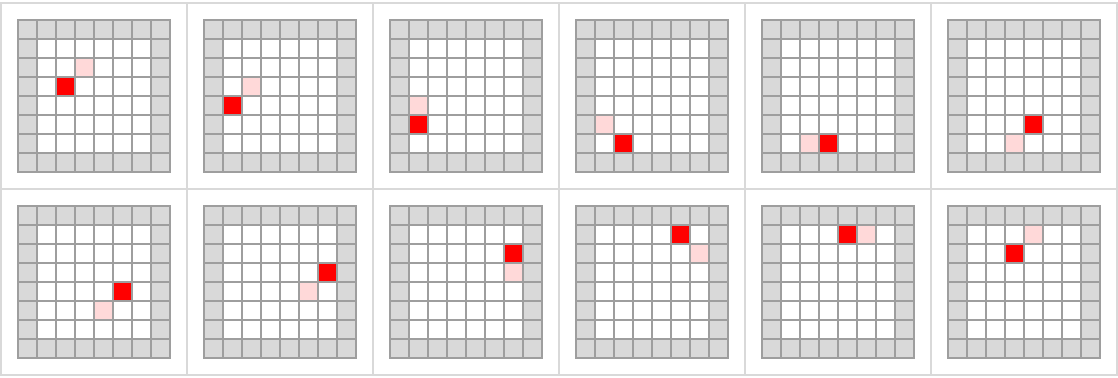Plot a block cellular automaton that seems to do something prime-like along its right edge:

 In:=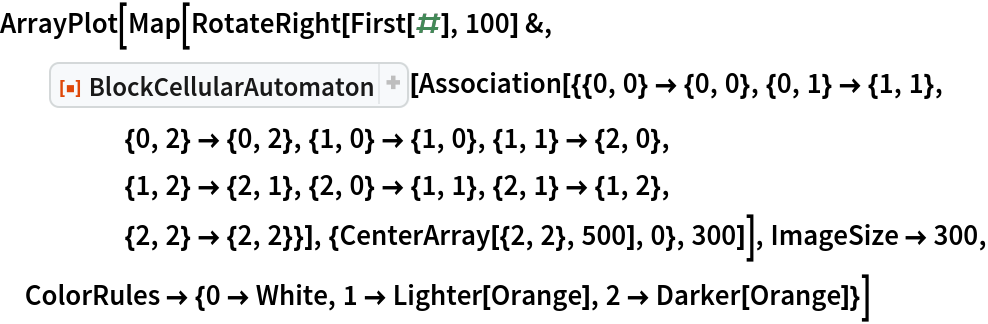Out=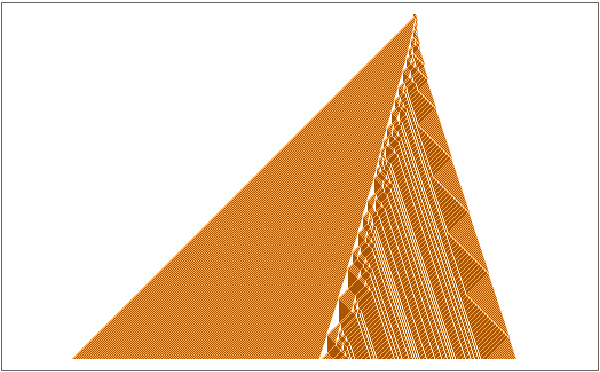Plot first differences of the semi-hypotenuse lengths:

 In:=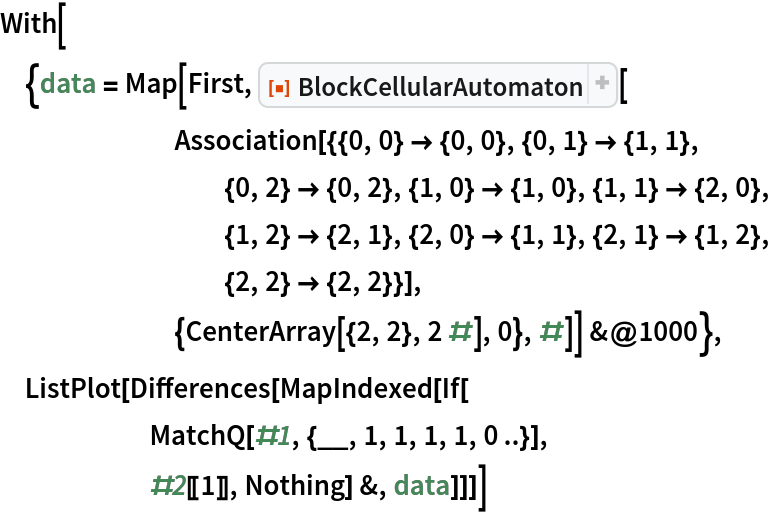Out=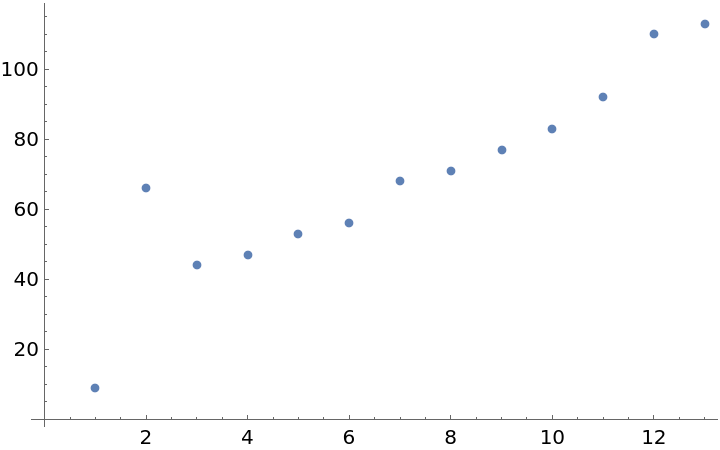Compare continuation of the integer sequence (not in OEIS!) with the Primes (the icon contains a 2 minute data run computed using the code above):

 In:=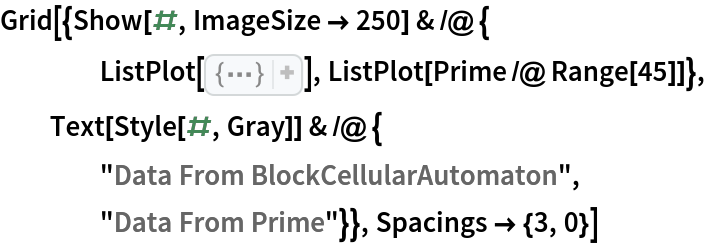Out=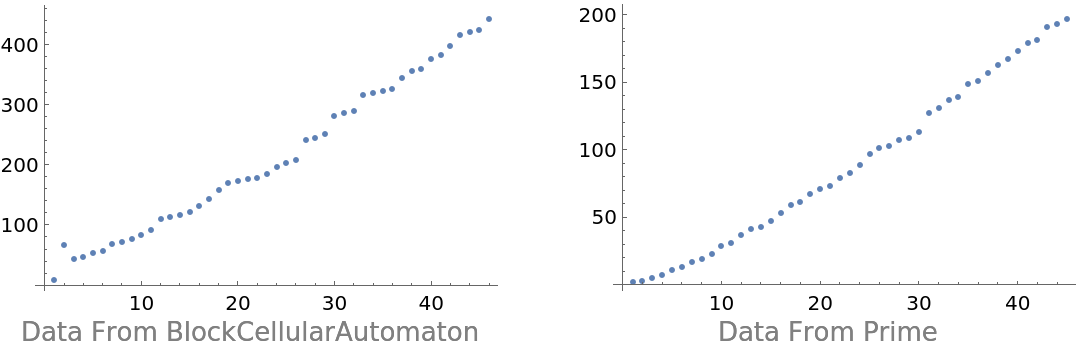## Version History

• 2.0.0 – 07 November 2022
• 1.0.0 – 12 August 2020